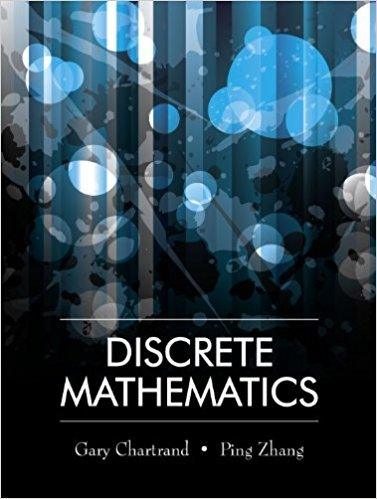×
×

# Solutions for Chapter 14.2: Coloring Graphs## Full solutions for Discrete Mathematics | 1st Edition

ISBN: 9781577667308Solutions for Chapter 14.2: Coloring Graphs

Solutions for Chapter 14.2
4 5 0 422 Reviews
14
2
##### ISBN: 9781577667308

Discrete Mathematics was written by and is associated to the ISBN: 9781577667308. This expansive textbook survival guide covers the following chapters and their solutions. Since 23 problems in chapter 14.2: Coloring Graphs have been answered, more than 12850 students have viewed full step-by-step solutions from this chapter. Chapter 14.2: Coloring Graphs includes 23 full step-by-step solutions. This textbook survival guide was created for the textbook: Discrete Mathematics, edition: 1.

Key Math Terms and definitions covered in this textbook
• Cayley-Hamilton Theorem.

peA) = det(A - AI) has peA) = zero matrix.

• Change of basis matrix M.

The old basis vectors v j are combinations L mij Wi of the new basis vectors. The coordinates of CI VI + ... + cnvn = dl wI + ... + dn Wn are related by d = M c. (For n = 2 set VI = mll WI +m21 W2, V2 = m12WI +m22w2.)

• Circulant matrix C.

Constant diagonals wrap around as in cyclic shift S. Every circulant is Col + CIS + ... + Cn_lSn - l . Cx = convolution c * x. Eigenvectors in F.

• Commuting matrices AB = BA.

If diagonalizable, they share n eigenvectors.

• Distributive Law

A(B + C) = AB + AC. Add then multiply, or mUltiply then add.

• Echelon matrix U.

The first nonzero entry (the pivot) in each row comes in a later column than the pivot in the previous row. All zero rows come last.

• Elimination matrix = Elementary matrix Eij.

The identity matrix with an extra -eij in the i, j entry (i #- j). Then Eij A subtracts eij times row j of A from row i.

• Identity matrix I (or In).

Diagonal entries = 1, off-diagonal entries = 0.

• Minimal polynomial of A.

The lowest degree polynomial with meA) = zero matrix. This is peA) = det(A - AI) if no eigenvalues are repeated; always meA) divides peA).

• Multiplication Ax

= Xl (column 1) + ... + xn(column n) = combination of columns.

• Normal equation AT Ax = ATb.

Gives the least squares solution to Ax = b if A has full rank n (independent columns). The equation says that (columns of A)·(b - Ax) = o.

• Normal matrix.

If N NT = NT N, then N has orthonormal (complex) eigenvectors.

• Pivot columns of A.

Columns that contain pivots after row reduction. These are not combinations of earlier columns. The pivot columns are a basis for the column space.

• Positive definite matrix A.

Symmetric matrix with positive eigenvalues and positive pivots. Definition: x T Ax > 0 unless x = O. Then A = LDLT with diag(D» O.

• Projection p = a(aTblaTa) onto the line through a.

P = aaT laTa has rank l.

• Rayleigh quotient q (x) = X T Ax I x T x for symmetric A: Amin < q (x) < Amax.

Those extremes are reached at the eigenvectors x for Amin(A) and Amax(A).

• Semidefinite matrix A.

(Positive) semidefinite: all x T Ax > 0, all A > 0; A = any RT R.

• Stiffness matrix

If x gives the movements of the nodes, K x gives the internal forces. K = ATe A where C has spring constants from Hooke's Law and Ax = stretching.

• Symmetric factorizations A = LDLT and A = QAQT.

Signs in A = signs in D.

• Vector space V.

Set of vectors such that all combinations cv + d w remain within V. Eight required rules are given in Section 3.1 for scalars c, d and vectors v, w.

×